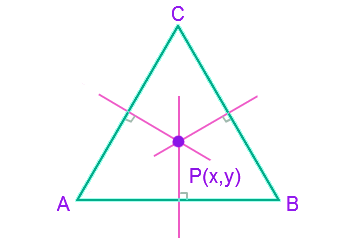# Circumcenter Formula

Circumcenter refers to the circumcenter of a triangle. The point where the perpendicular bisectors of a triangle meets. It lies inside for an acute, outside for an obtuse and at the center of the hypotenuse for the right triangle.Method to Calculate the Circumcenter of aTriangle

The steps to find the circumcenter of a triangle:

• Find and Calculate the midpoint of given coordinates or midpoints (AB, AC, BC)
• Calculate the slope of the particular line
• By using the midpoint and the slope, find out the equation of line (y-y1) = m (x-x1)
• Find out the other line of equation in the similar manner
• Solve the two bisector equation by finding out the intersection point
• Calculated intersection point will be the circumcenter of the given triangle

### Solved Examples

Question 1: If three coordinates of a triangle are (3,2), (1,4), (5,4). Calculate the circumcenter of this triangle ?

Solution:

Given points are,
A = (3, 2), B = (1, 4), C = (5, 4)
To find out the circumcenter we have to solve any two bisector equations and find out the intersection points.

So, mid point of AB = ($\frac{3+1}{2}$,$\frac{2+4}{2}$) = (2,3)

Slope of AB = ($\frac{4-2}{1-3}$) = -1
Slope of the bisector is the negative reciprocal of the given slope.
So, the slope of the perpendicular bisector = 1
Equation of AB with slope 1 and the coordinates (2,3) is,
(y – 3) = 1(x – 2)
x – y = -1………………(1)
Similarly, for AC
Mid point of AC = ($\frac{3+5}{2}$,$\frac{2+4}{2}$) = (4,3)

Slope of AC = ($\frac{4-2}{5-3}$) = 1
Slope of the bisector is the negative reciprocal of the given slope.
So, the slope of the perpendicular bisector = -1
Equation of AC with slope -1 and the coordinates (4,3) is,
(y – 3) = -1(x – 4)
y – 3 = -x + 4
x + y = 7………………(2)
By solving equation (1) and (2),
(1) + (2) ⇒ 2x = 6; x = 3
Substitute the value of x in to (1)
3 – y = -1
y = 3 + 1 = 4
So the circumcenter is (3, 4)